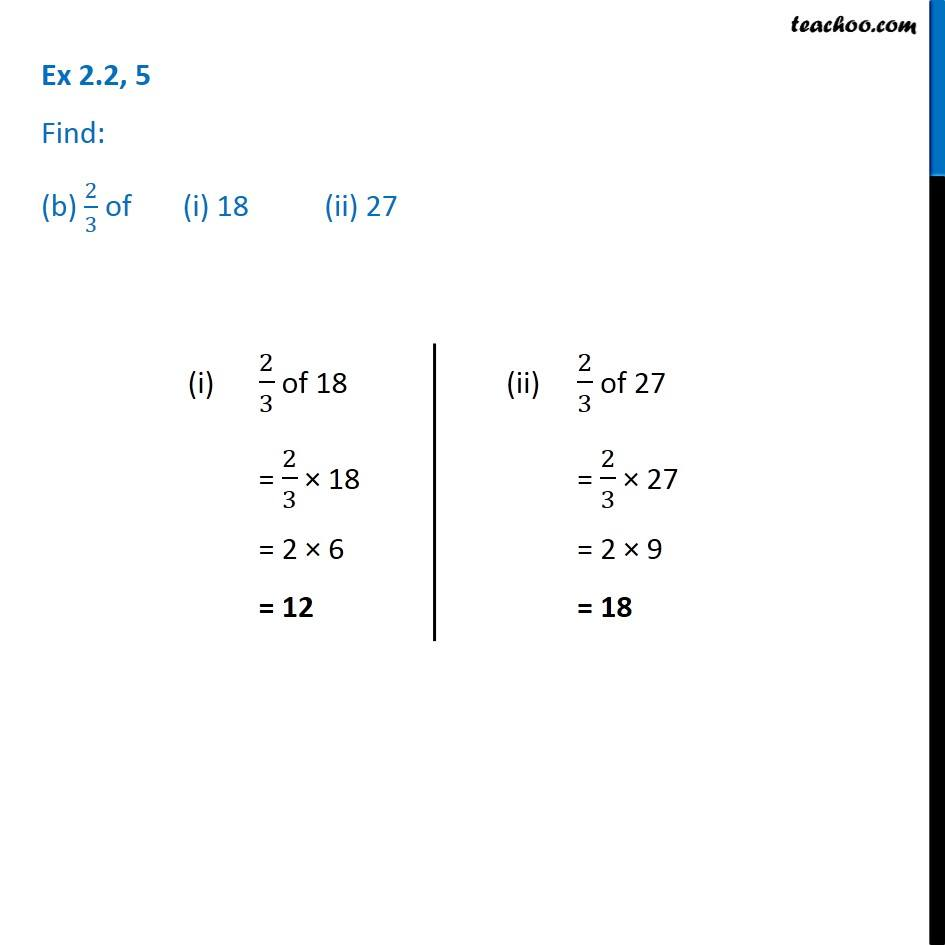1. Chapter 2 Class 7 Fractions and Decimals
2. Serial order wise
3. Ex 2.2

Transcript

Ex 2.2, 5 Find: (b) 2/3 of (i) 18 (ii) 27 (i) 2/3 of 18 = 2/3 × 18 = 2 × 6 = 12 (ii) 2/3 of 27 = 2/3 × 27 = 2 × 9 = 18